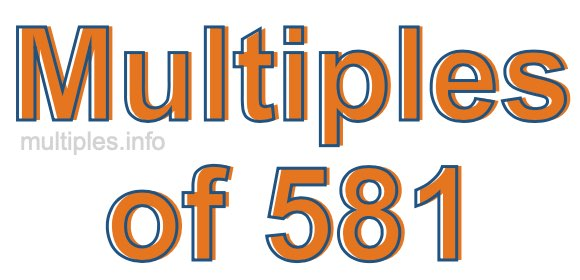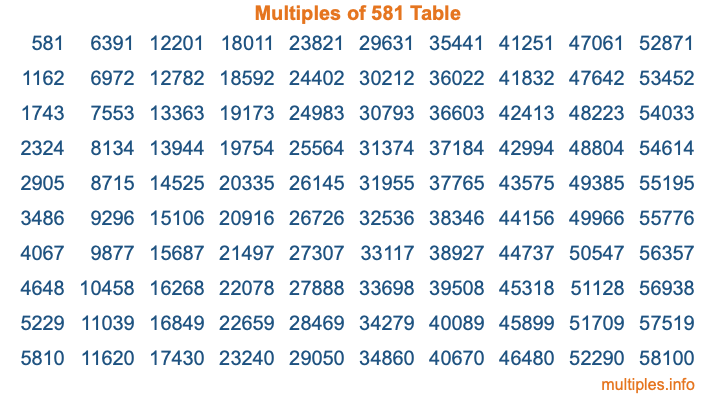Multiples of 581Welcome to the Multiples of 581 page. Here we will first teach you everything you will ever need to know about the multiples of 581, and then give you a study guide summary of everything we taught you to make sure you remember it all. Use this page to look up facts and learn information about the multiples of 581. This page will make you a multiples of five hundred eighty-one expert!

Definition of Multiples of 581
Multiples of 581 are all the numbers that when divided by 581 equal an integer. Each of the multiples of 581 are called a multiple. A multiple of 581 is created by multiplying 581 by an integer.

Therefore, to create a list of multiples of 581, you start with 1 multiplied by 581, then 2 multiplied by 581, then 3 multiplied by 581, and so on for as long as you want. Thus, the list of the first five multiples of 581 is 581, 1162, 1743, 2324, and 2905. To see a larger list of multiples of 581, see the printable image of Multiples of 581 further down on this page. We also have a category where you can choose any nth multiple of 581.

Multiples of 581 Checker
The Multiples of 581 Checker below checks to see if any number of your choice is a multiple of 581. In other words, it checks to see if there is any number (integer) that when multiplied by 581 will equal your number. To do that, we divide your number by 581. If the the quotient is an integer, then your number is a multiple of 581.

Is  a multiple of 581?

Least Common Multiple of 581 and ...
A Least Common Multiple (LCM) is the lowest multiple that two or more numbers have in common. This is also called the smallest common multiple or lowest common multiple and is useful to know when you are adding our subtracting fractions. Enter one or more numbers below (581 is already entered) to find the LCM.

Check out our LCM Calculator if you need more details about the Least Common Multiple or if you need the LCM for different numbers for adding and subtraction fractions.

nth Multiple of 581
As we stated above, 581 is the first multiple of 581, 1162 is the second multiple of 581, 1743 is the third multiple of 581, and so on. Enter a number below to find the nth multiple of 581.

th multiple of 581

Multiples of 581 vs Factors of 581
581 is a multiple of 581 and a factor of 581, but that is where the similarities end. All postive multiples of 581 are 581 or greater than 581. All positive factors of 581 are 581 or less than 581.

Below is the beginning list of multiples of 581 and the factors of 581 so you can compare:

Multiples of 581: 581, 1162, 1743, 2324, 2905, etc.

Factors of 581: 1, 7, 83, 581

As you can see, the multiples of 581 are all the numbers that you can divide by 581 to get a whole number. The factors of 581, on the other hand, are all the whole numbers that you can multiply by another whole number to get 581.

It's also interesting to note that if a number (x) is a factor of 581, then 581 will also be a multiple of that number (x).

Multiples of 581 vs Divisors of 581
The divisors of 581 are all the integers that 581 can be divided by evenly. Below is a list of the divisors of 581.

Divisors of 581: 1, 7, 83, 581

The interesting thing to note here is that if you take any multiple of 581 and divide it by a divisor of 581, you will see that the quotient is an integer.

Multiples of 581 Table
Below is an image of the first 100 multiples of 581 in a table. The table is in chronological order, column by column. The first column has the first ten multiples of 581, the second column has the next ten multiples of 581, and so on.The Multiples of 581 Table is also referred to as the 581 Times Table or Times Table of 581. You are welcome to print out our table for your studies.

Negative Multiples of 581
Although not often discussed or needed in math, it is worth mentioning that you can make a list of negative multiples of 581 by multiplying 581 by -1, then by -2, then by -3, and so on, to get the following list of negative multiples of 581:

-581, -1162, -1743, -2324, -2905, etc.

Multiples of 581 Summary
Below is a summary of important Multiples of 581 facts that we have discussed on this page. To retain the knowledge on this page, we recommend that you read through the summary and explain to yourself or a study partner why they hold true.

There are an infinite number of multiples of 581.

A multiple of 581 divided by 581 will equal a whole number.

581 divided by a factor of 581 equals a divisor of 581.

The nth multiple of 581 is n times 581.

The largest factor of 581 is equal to the first positive multiple of 581.

581 is a multiple of every factor of 581.

581 is a multiple of 581.

A multiple of 581 divided by a divisor of 581 equals an integer.

581 divided by a divisor of 581 equals a factor of 581.

Any integer times 581 will equal a multiple of 581.

Multiples of a Number
Here you can get the multiples of another number, all with the same attention to detail as we did for multiples of 581 on this page.

Multiples of
Multiples of 582
Did you find our page about multiples of five hundred eighty-one educational? Do you want more knowledge? Check out the multiples of the next number on our list!